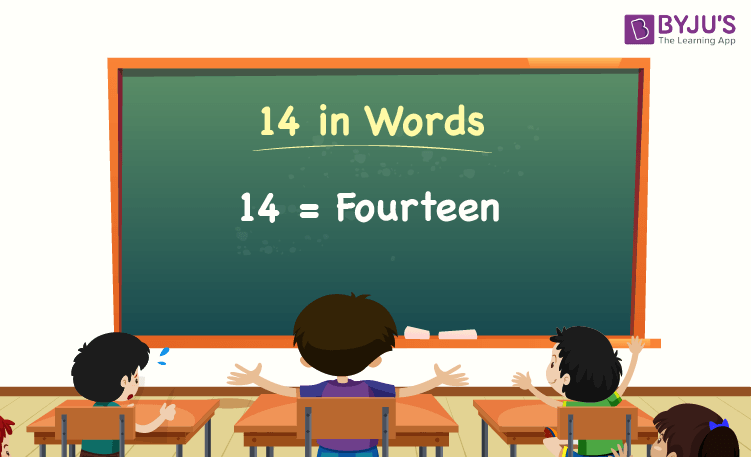# 14 in Words

14 in words is Fourteen. For example, if you spent Rs. 14 in a day, you can write, “I have spent Rs. Fourteen in a day”. The place value chart helps to write the number name of 14.

 14 in Words: Fourteen

In this article, we will discuss how to write the number 14 in words and look at many solved examples in detail.

## How to Write 14 in Words?The number 14 in words is written using the place value of a number. Thus, to write 14 in words, find the place value of each digit of 14.

For a number 14,

The digit in unit/one’s place = 4

The digit in ten’s place = 1

Since the number 14 is a two-digit number, there are no hundreds and thousands.

Thus, the place value chart for 14 is:

 Tens Ones 1 4

Therefore, the number 14 in words is Fourteen.

### Examples

Example 1:

Express the value of 10 + 4 in words.

Solution:

Given expression: 10 + 4

⇒ 10 + 4 = 14

So, the value of 10 + 4 is 14.

Hence, 14 in words is fourteen.

Example 2:

Find the value of fourteen plus twenty, and express it in words.

Solution:

Fourteen = 14

Twenty = 20

Fourteen plus twenty = 14 + 20 = 34

Hence, the value of fourteen plus twenty is thirty-four in words.

Download BYJU’S – The Learning App, and learn all Maths-related concepts easily by exploring more learning videos.

## Frequently Asked Questions on 14 in Words

Q1

### Write 14 in words.

14 in words is fourteen.

Q2

### Find the value of 20 – 6, and express the answer in words.

Simplifying 20 – 6, we get 14. Hence, 14 in words is fourteen.

Q3

### How to write fourteen in numbers?

Fourteen in numbers is 14.

Q4

### Is 14 an even number?

Yes, 14 is an even number.

Q5

### Is 14 a perfect square number?

No, 14 is not a perfect square number.

Test your Knowledge on 14 in Words